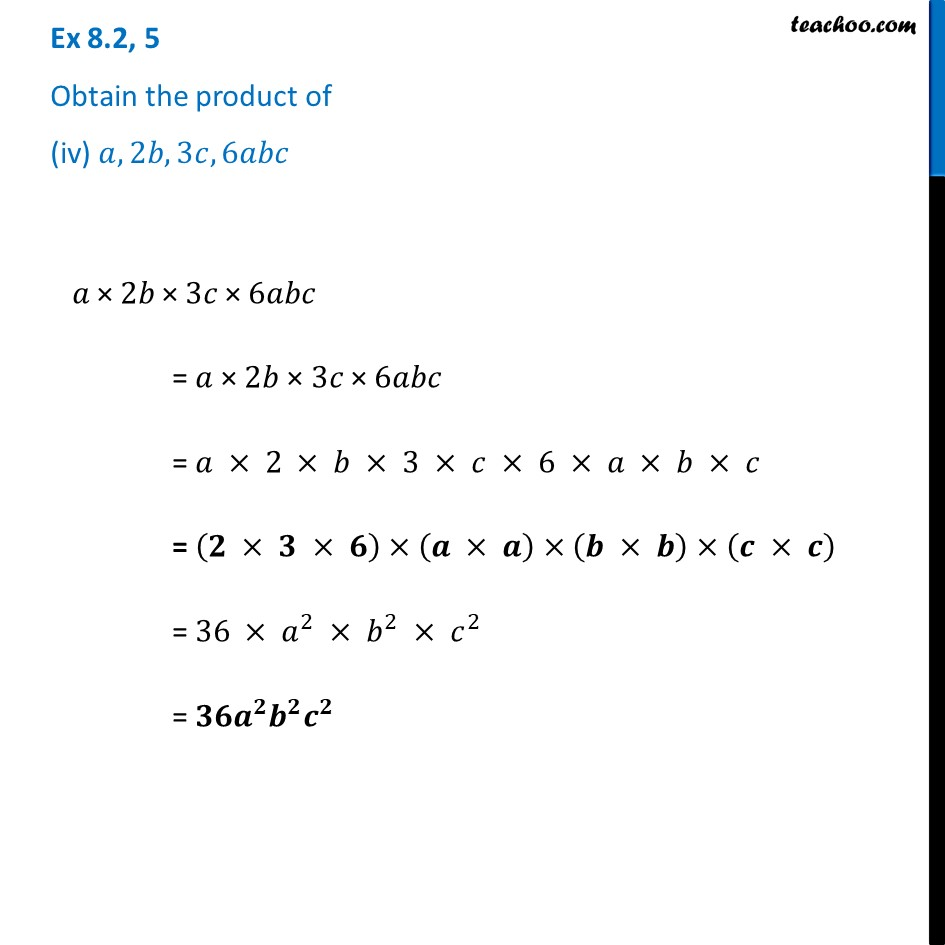Ex 8.2

Chapter 8 Class 8 Algebraic Expressions and Identities
Serial order wiseLearn in your speed, with individual attention - Teachoo Maths 1-on-1 Class

### Transcript

Ex 8.2, 5 Obtain the product of (iv) 𝑎, 2𝑏, 3𝑐, 6𝑎𝑏𝑐 𝑎" ×" 2𝑏" × " 3𝑐" × " 6𝑎𝑏𝑐 = 𝑎 × 2𝑏 × 3𝑐 × 6𝑎𝑏𝑐 = 𝑎 × 2 × 𝑏 × 3 × 𝑐 × 6 × 𝑎 × 𝑏 × 𝑐 = (𝟐 × 𝟑 × 𝟔)×(𝒂 × 𝒂)×(𝒃 × 𝒃)×(𝒄 × 𝒄) = 36 × 𝑎^2 × 𝑏^2 × 𝑐^2 = 𝟑𝟔𝒂^𝟐 𝒃^𝟐 𝒄^𝟐